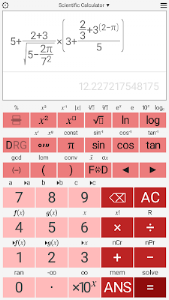# Basic Scientific Calculator v1.4.0

Free

### Basic Scientific Calculator / Screenshots### Basic Scientific Calculator / Description

An ad free version of the Basic Scientific Calculator is also available.

• Easy to use interface allows the calculation of fractions and decimals.

• The text-book style display allows you to enter calculations as they would be written.

• Calculate square roots powers and percentages.

• Conversion between decimal and surd answers.

• logarithms base 10 e (natural logarithm) and n.

• trigonometric functions and their inverses.

• 26 scientific constants.

• unit conversions.

• factorials combinations and permutations.

• Previous 10 calculations stored and re-editable.

• The calculator's colors are customizable.

• User definable decimal marker (point or comma).

• Optional thousands separator. Choose between space or comma/point(depends on decimal marker).

• Variable precision up to 15 significant figures.